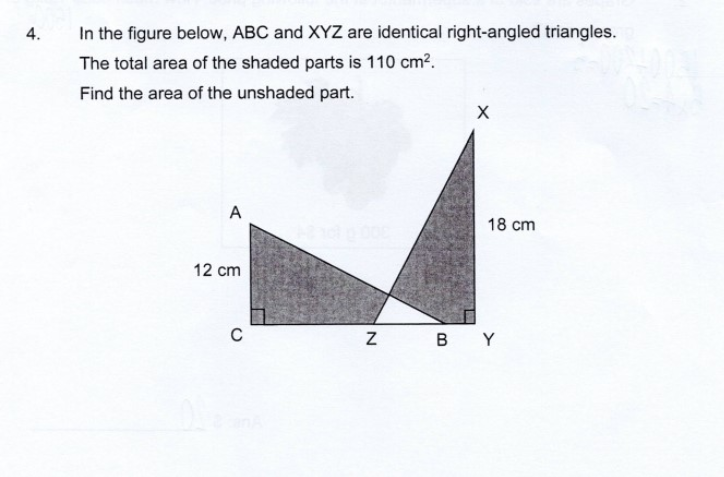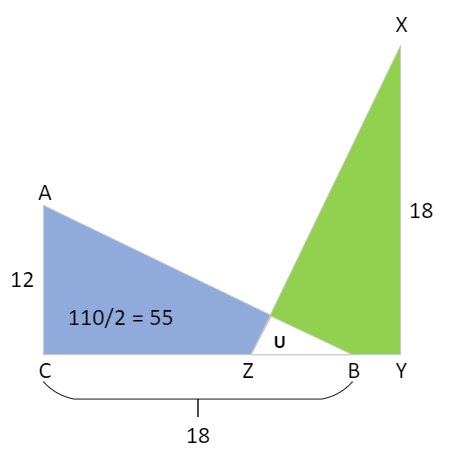# QuestionIn the figure below,ABC and XYZ are identical right-angled triangles.The total area of the shaded parts is 110cm2.Find the area of the unshaded part.

How to tackle this question?

` ````Let U be Unshaded area. Blue area = Area ABC - U Green area = Area XYZ - U But Area ABC = Area XYZ So Blue area = Green area Thus Blue area = 110/2 = 55cm2 and U = Area ABC - Blue area = (18 x 12)/2 - 55 = 53cm2 The key is to realize that the shaded parts are equal as the same U is cut from ABC and XYZ.```
` `
0 Replies 1 Like

If ABC and XYZ are identical triangle, then length XY = length CB = 18cm

And length AC = length ZY = 12cm

Area of triangle ABC = Area of triangle XYZ = 18*12/2= 108 cm square